Courses

# Frequency Domain Analysis of Control Systems - 3

## 20 Questions MCQ Test Topicwise Question Bank for Electrical Engineering | Frequency Domain Analysis of Control Systems - 3

Description
This mock test of Frequency Domain Analysis of Control Systems - 3 for Electrical Engineering (EE) helps you for every Electrical Engineering (EE) entrance exam. This contains 20 Multiple Choice Questions for Electrical Engineering (EE) Frequency Domain Analysis of Control Systems - 3 (mcq) to study with solutions a complete question bank. The solved questions answers in this Frequency Domain Analysis of Control Systems - 3 quiz give you a good mix of easy questions and tough questions. Electrical Engineering (EE) students definitely take this Frequency Domain Analysis of Control Systems - 3 exercise for a better result in the exam. You can find other Frequency Domain Analysis of Control Systems - 3 extra questions, long questions & short questions for Electrical Engineering (EE) on EduRev as well by searching above.
QUESTION: 1

Solution:
QUESTION: 2

### The Bode plot for a transfer function is shown below: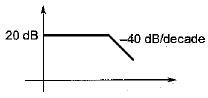The steady state error corresponding to a parabolic input is

Solution:

Initial slope of the system = 0 dB/decade
Therefore, given system is a type-0 system.
For type-0 system, for parabolic input, steady state error is infinite since,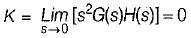QUESTION: 3

### The frequency at which the Nyquist plot crosses the negative real axis is known as

Solution:

At ω = ωgc, ϕ = -180°
i.e. Nyquist plot crosses the negative real axis.

QUESTION: 4

A unity feedback system has a forward path gain of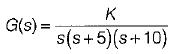The phase angle of the Nyquist plot for ω → ∞ is

Solution: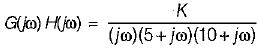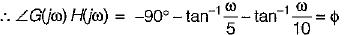At ω → ∞, ϕ = -90° - 90° - 90° = -270° = +90°

QUESTION: 5

The open loop transfer function of a system is given as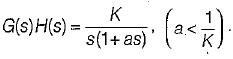The Bode plot of this system is represented as:

Solution: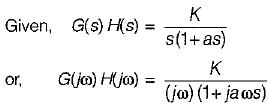Initial slope = -20 dB/decade
= -6 dB/octave (Since Type = 1)
Corner frequency is,
ω= (1/a) rad/sec
Initial slope = -20 dB/decade
= -6 dB/octave (Since Type = 1)
Corner frequency is,
ωc = 1/a rad/sec
It will cross 0 dB line at ω = K and K < 1/a.
So, the Bode plot will be as shown below.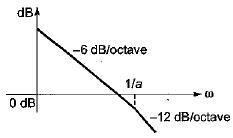QUESTION: 6

A second order system has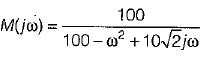It’s Mp (peak magnitude) will be approximately

Solution: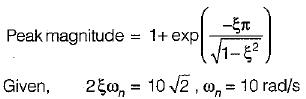∴ ξ = 1/√2
So, peak magnitude = 1 + e-π ≈ 1

QUESTION: 7

The closed loop transfer function of a control system is given by
C(s)/R(s) = 1/(1+s)
For the input r(t) = sint, the steady state value of c(t) is equal to

Solution:

Given,
C(s)/R(s) = 1/(1+s)
Thus,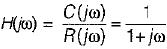Here,
ω = 1 rad/s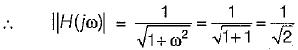and  ∠H(jω) = -tan-1ω = -tan-1(1)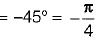∴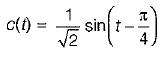QUESTION: 8

A system has transfer function equal to (1-s)/(1+s). Its gain at ω = 1 rad/s is

Solution:

Given transfer function represents an all pass filter which has gain of 1 at all frequencies.

QUESTION: 9

The gain margin of a unity negative feedback system having forward path transfer function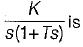Solution: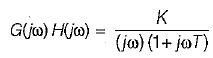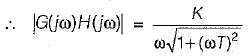∠G(jω) H(jω) = -90° - tan-1ωT = ϕ
At ω = ωpc,
ϕ = -180º
∴ -90° - tan-1ωpc = -180° or, ωpc = ∞ rad/s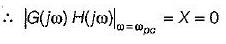∴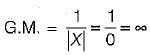QUESTION: 10

The characteristic equation of a closed loop control system is given by s2 + 4s + 16 = 0. The resonant frequency (in radian/sec) of the system is

Solution:

Given, s2 + 4s+ 16 = 0
or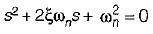∴ ωn = 4 rad/s
and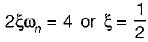∴ Resonant frequency,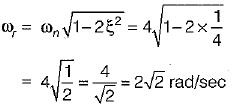QUESTION: 11

The gain margin (in dB) of a system having the open-loop transfer function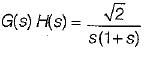is

Solution:

Given,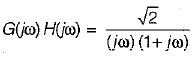∴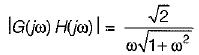and ∠G{jω)H(jω) = -90° - tan-1 ω = ϕ
At ω = ωpc, ϕ = -180°
∴ -90° - tan-1 ωpc = -180° or ωpc = ∞
∴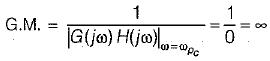QUESTION: 12

Nichol’s chart is useful for detailed study and analysis of

Solution:

Nichol’s chart consists of magnitude and phase angle of a closed loop system represented as a family of circle known as M and N-circle.

QUESTION: 13

The forward path transfer function of an unity feedback system is given by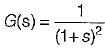What is the phase margin for this system?

Solution:

Given,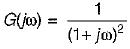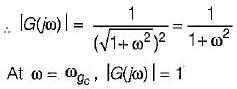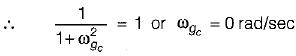Now,
∠G(jω) = -2tan-1ω = ϕ
At
ω = ωgc = 0 rad/s
ϕ = -2 x 0 = 0º
∴   P.M. = 180° + ϕ
= 180° + 0° = 180° = π

QUESTION: 14

The closed loop system having the open loop transfer function,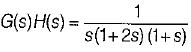is

Solution:

Given,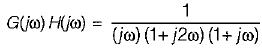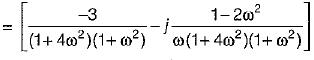For finding ωgc, Img[G(jω)H(jω)] = 0
or,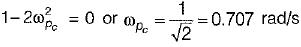Now,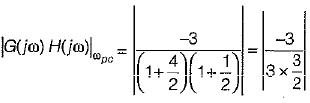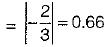Thus, Nyquist plot will cut real axis at -0.66.
The given system: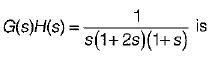of type-1 and order-3.
Therefore, Nyquist plot will be as shown below: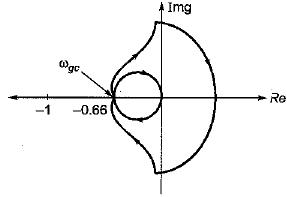Now, from above Nyquist plot,
N = 0 = Number of encirclements.
Also, P = number of open loop poles in RH s-plane = 0
Now, N = P-Z
or, 0 = 0 - Z or Z = 0
Thus, no. of closed loop poles in RH s-plane = 0.
Hence, system is stable.

QUESTION: 15

The nyquist plot for a unity feedback control system having open loop transfer function G(s) = K(1-s)/(s+1) is shown in figure below :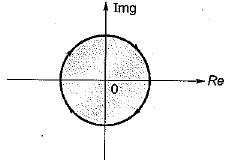The system is

Solution:

Given,
G(s)H(s) = K(1-s)/(s+1)
or,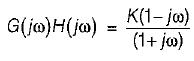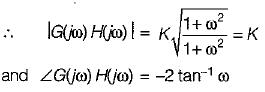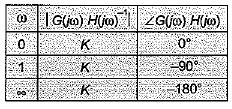Thus, the radius of Nyquist plot will be K.
Now, no.of open loop poles in RH s-piane = 0.
When K > 1, no. of encirclement, N = -1
When K< 1, no. of encirclement, N = 0
Thus, for K > 1, N = P - Z
or,    -1 = 0 - Z or Z = 1
∴ System is unstable.
For K < 1, N = P - Z or 0 = 0 - Z or Z = 0
∴ System is stable..

QUESTION: 16

A unity feedback control system has a forward path transfer function of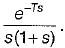Its phase value will be zero at a frequency of ω1.
Which one of the following equation should be satisfied for it?

Solution:

Given,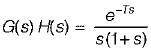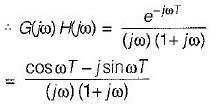or, ∠G(jω) H(jω) = -ωT - 90° - tan-1 ω
At ω = ω1, ∠G(jω) H(jω) = 0°
∴ -ω1 T-90° -tan-1ω1 = 0
or, tan-1ω= - (ω1T + 90°)
or, ω1, = -tan (90° + ω1T) = cot ω1T
∴ ω1 = cot(ω1T)

QUESTION: 17

What is the approximate value of gain margin in the Nyquist diagram shown below?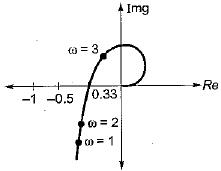Solution:

At ω = ωgc,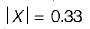(Using Nyquist diagram)
∴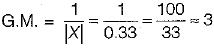QUESTION: 18

The magnitude-frequency response of a control system is given in figure below: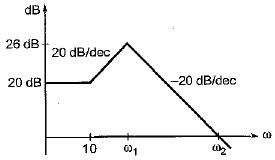The values of ω1 and ω2 are respectively

Solution:

Number of decade change from first break frequency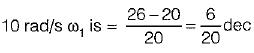Now,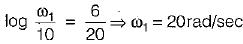Now, no. of decade change from break frequency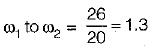∴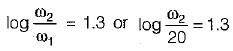or,
ω2 = 400 rad/s

QUESTION: 19

Match List-I with List-Il and select the correct answer using the codes given below the lists:
List-I
A. Resonant peak
B. Damped natural frequency (rad/s)
C. Resonant frequency (rad/s)
D. Peak overshoot
List - II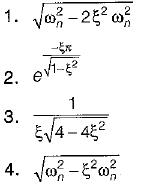Codes:
A B C D
(a) 3 4 1 2
(b) 3 1 4 2
(c) 2 4 1 3
(d) 2 1 4 3

Solution:
QUESTION: 20

A second order underdamped system has a damping ratio of 0.8. It is subjected to a sinusoidal input of unit amplitude. It has resonant peak of

Solution:

Resonant frequency is given by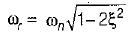For resonant peak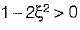or,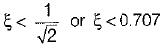Since ξ > 0.707, therefore there is no Mr (resonant peak).